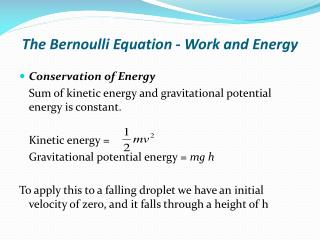Download PresentationThe Bernoulli Equation - Work and Energy

Loading in 2 Seconds...

# The Bernoulli Equation - Work and Energy - PowerPoint PPT Presentation

The Bernoulli Equation - Work and Energy. Conservation of Energy Sum of kinetic energy and gravitational potential energy is constant. Kinetic energy = Gravitational potential energy = mg hI am the owner, or an agent authorized to act on behalf of the owner, of the copyrighted work described.
Download Presentation## The Bernoulli Equation - Work and Energy

An Image/Link below is provided (as is) to download presentation

Download Policy: Content on the Website is provided to you AS IS for your information and personal use and may not be sold / licensed / shared on other websites without getting consent from its author.While downloading, if for some reason you are not able to download a presentation, the publisher may have deleted the file from their server.

- - - - - - - - - - - - - - - - - - - - - - - - - - E N D - - - - - - - - - - - - - - - - - - - - - - - - - -
Presentation Transcript
1. The Bernoulli Equation - Work and Energy • Conservation of Energy Sum of kinetic energy and gravitational potential energy is constant. Kinetic energy = Gravitational potential energy = mg h To apply this to a falling droplet we have an initial velocity of zero, and it falls through a height of h

2. Initial kinetic energy = 0 • Initial potential energy = mg h • Final kinetic energy = • Final potential energy = 0 • Notice that the pressure is constant. Initial kinetic energy + Initial potential energy = Final kinetic energy + Final potential energy

3. In this way , the velocity of a drop of water can calculated in theory. It is also applied to any case. For example to calculate the velocity of water exiting an orifice: Initial kinetic energy = 0 Initial potential energy = mg z1 Final kinetic energy = 0.5 mu2 Final potential energy = mgz2

4. We know that kinetic energy + potential energy = constant Compare these equations

5. Bernoulli’s Equation Bernoulli’s equation is one of the most important and useful equations in fluid mechanics. It may be written, We see that from applying equal pressure or zero velocities we get the two equations from the section above. They are both just special cases of Bernoulli’s equation.

6. Bernoulli’s equation has some restrictions in its applicability, they are: - Flow is steady; - Density is constant (which also means the fluid is incompressible); - Friction losses are negligible. • All these conditions are impossible to satisfy at any instant in time! Fortunately for many real situations where the conditions are approximately satisfied, the equation gives very good results.

7. By the principle of conservation of energy the total energy in the system does not change, Thus the total head does not change. So the Bernoulli equation can be written,

8. An example of the use of the Bernoulli equation. When the Bernoulli equation is combined with the continuity equation the two can be used to find velocities and pressures at points in the flow

9. A fluid of constant density ρ = 960 kg / m3 is flowing steadily through the above tube. The diameters at the sections are d1 = 100 mm and d2 = 80 mm. The gauge pressure at 1 is p1 = 200 kN/ m2and the velocity here is u1 = 5 m/ s. We want to know the gauge pressure at section 2. • The tube is horizontal, with z1 = z2 so Bernoulli gives us the following equation for pressure at section 2:

10. But we do not know the value of u2 . We can calculate this from the continuity equation: Discharge into the tube is equal to the discharge out i.e. A1u1 = A2u2 u2 = 7.8125 m/s p2 = 217.3 kN/m2

11. Notice the figure and relate with Bernoulli’s equation

12. Energy losses due to friction

13. In a real pipe line there are energy losses due to friction - these must be taken into account as they can be very significant. The total head - or total energy per unit weight – is constant. We are considering energy conservation, so if we allow for an amount of energy to be lost due to friction the total head will change.